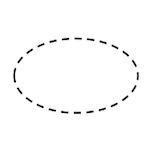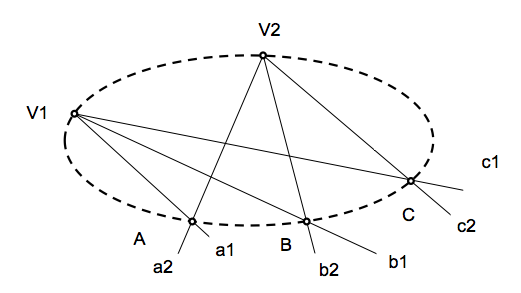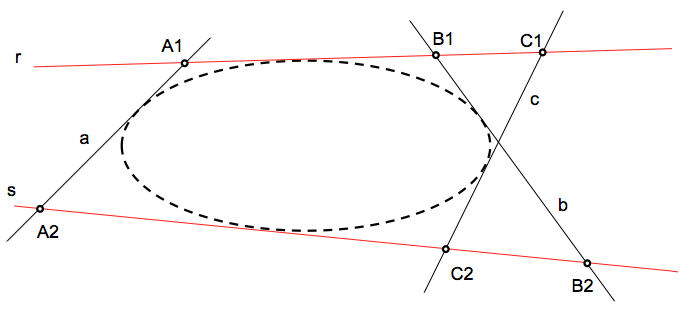# Geometría proyectiva: Definition of the conical projectiveConic curves, further treatment of the metric based on the notions of tangency, have a projective treatment that relies on the concepts of series and do projective.

We will see two definitions of conic adapted to “World points” o al “world of straight” according to the interest, in what is defined as the definitions “point” or “tangential” of conic curves.

The precise definition or tangential taper treatment allow us the projective, providing tools and projective as the center axis for determining new points and tangent to the same.

### Series of second order

The common points of two coplanar beams straight, projective each other, determine a number of points based on a second order conic curve called projective point.

This set of points will call you “series of second order”, being kissed conical geometry of this new. (see the parallel with the line in the series of first-order)Data two beams projective lines together, of vertices V1 V2 are, conic curve will call the locus of intersection points of each pair of homologous rays (a1-a2) of these beams.

### Haces of second order

We can do dual definitions from the specific model described in the previous section.
The two coplanar lines common series of straight, projective each other, determine a second straight beam by a curved base called projective tangent cone.

This set of lines joining pairs of homologous points in two series you will call proyecticas “beam of second order”. The cone is the basis of this beam, infinite elements being tangent to this basis we can get.Given of series projective points of each other, de bases r and s, conic curve will call the envelope locus straight projection (containing) of each pair of homologous points (A1-A2) this series.